# Legendre Polynomials

(redirected from Angular degree)

## Legendre polynomials

[lə′zhän·drə ‚päl·i′nō·mē·əlz]
(mathematics)
A collection of orthogonal polynomials which provide solutions to the Legendre equation for nonnegative integral values of the parameter.
McGraw-Hill Dictionary of Scientific & Technical Terms, 6E, Copyright © 2003 by The McGraw-Hill Companies, Inc.
The following article is from The Great Soviet Encyclopedia (1979). It might be outdated or ideologically biased.

## Legendre Polynomials

a system of polynomials of successively increasing degree. The polynomials were first investigated by A. Legendre and P. Laplace independently of each other between 1782 and 1785. For n = 0, 1, 2, …, the Legendre polynomials Pn(x) can be defined by the formula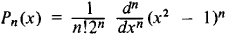The first few polynomials are

p0 (x) = 1

p1 (x) = x

p2 (x) = 1/2(3x2 - 1)

p3 (x) = 1/2(5x2 - 3x)

p4 (x) = 1/8(35x4 - 30x2 + 3)

p5 (x) = 1/8(63x5 - 70x3 + 15x)

All the zeros of P n (x) are real, lie in the interval [–1, + 1], and alternate with the zeros of Pn + 1 (x). The Legendre polynomials are a complete set of orthogonal polynomials on the interval [–1, + 1]. Thus, it is possible to expand an arbitrary function /(jc) integrable over the interval [– 1, +1] in a series of Legendre polynomials: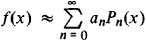where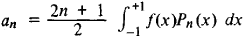The type of convergence of this series is roughly the same as that of a Fourier series. The Legendre polynomials are given explicitly by the formula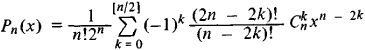The generating function is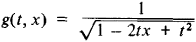that is, the Legendre polynomials are the coefficients in the expansion of this function in powers of t. They are recursively defined by

nPn (x) + (n - 1)Pn-2 (x) - (2n - 1)xPn-1 (x) = 0

Pn (x)satisfies the differential equation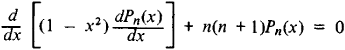which arises when separating the variables in Laplace’s equation in spherical coordinates.

### REFERENCES

Janke, E., F. Emde, and F. Lösch. Spetsial’nye funktsii; grafiki, tablitsy, 2nd ed. Moscow, 1968. (Translated from German.)
Lebedev, N. N. Spetsial’nye funktsii i ikh prilozheniia, 2nd ed. MoscowLeningrad, 1963.

V. I. BITIUTSKOV